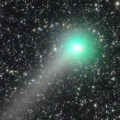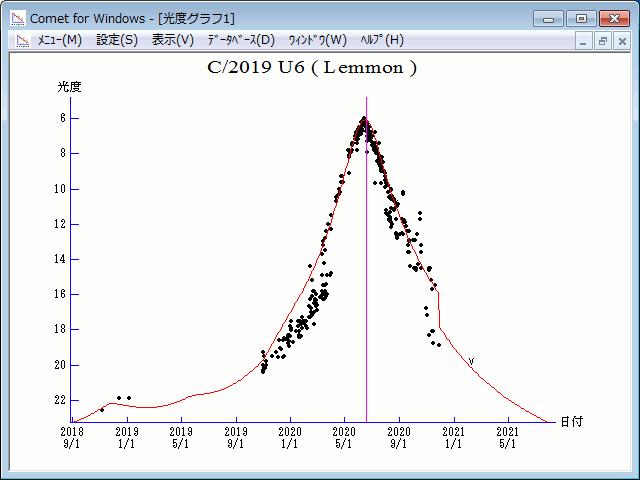# \$B%l%b%sWB@1(B

C/2019 U6 ( Lemmon )###\$B%W%m%U%#!<%k(B

 \$BH/8+F|(B 2019\$BG/(B10\$B7n(B31\$BF|(B \$BH/8+8wEY(B 20.5\$BEy(B \$BH/8+ R. A. Kowalski (Mt. Lemmon Survey)

###\$B###\$B50F;MWAG(B

```Epoch 2020 May 31.0 TT = JDT 2459000.5
T 2020 June 18.82214 TT                                 Rudenko
q   0.9142396            (2000.0)            P               Q
z  +0.0023143      Peri.  329.66104     -0.68858485     +0.06105880
+/-0.0000013      Node   235.70576     -0.35185738     -0.89942162
e   0.9978842      Incl.   61.00132     -0.63407199     +0.43279623
From 362 observations 2018 Nov. 6-2020 May 29, mean residual 0".7.
```

###\$B@1?^(B###\$B8wEYJQ2=(B

```        H = 14.0  G = 0.15                       [    ,-231]  (              \$B!A(B2019\$BG/(B10\$B7n(B31\$BF|(B)
m1 = 7.0 + 5 log\$B&\$(B + 20.0 log r(t + 10)  [-231,    ]  (2019\$BG/(B10\$B7n(B31\$BF|!A(B              )
```##### \$B50F;MWAG\$O(BM.P.E.C. 2020-L06\$B\$K7G:\\$5\$l\$?\$b\$N\$G\$9!#(B \$B@1?^\$O%9%F%i%J%S%2!<%?(B Ver.10 (\$B%"%9%H%m%"!<%D(B) \$B\$G:n@.\$7\$?\$b\$N\$G\$9!#(B \$B8wEY%0%i%U\$O(BComet for Windows\$B\$G:n@.\$7\$?\$b\$N\$G\$9!#(B